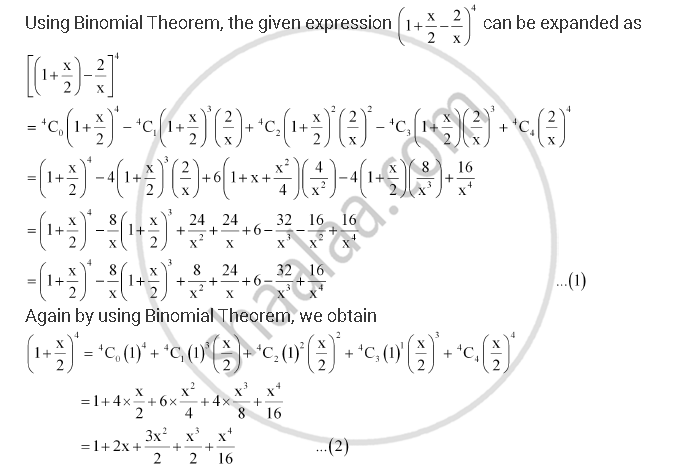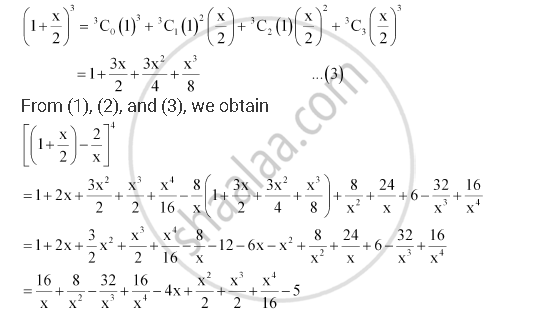# Expand Using Binomial Theorem (1+ X/2 - 2/X)^4, X != 0 - Mathematics

Expand using Binomial Theorem (1+ x/2 - 2/x)^4, x != 0

#### SolutionConcept: Binomial Theorem for Positive Integral Indices
Is there an error in this question or solution?

#### APPEARS IN

NCERT Class 11 Mathematics
Chapter 8 Binomial Theorem
Q 9 | Page 176